## Determining Power Law parameter(s) using Bayesian modeling with PyMC

### Model declaration

To begin with, I define the model that we want to use for our Bayesian inference approach. As the power law distribution is not already included in the PyMC library, we have to define it ourself. For doing so, I resort to the probability density and mass functions defined by Clauset et al. in http://arxiv.org/abs/0706.1062.

We have to differ between continiuous and discrete distributions.

For the continuious case we use the following definition:

$p(x) = C * f(x) = (\alpha-1)*x_{min}^{\alpha-1} * x^{-\alpha}$

And for the discrete the following where $\zeta$ is the Hurwitz zeta function:

$p(x) = C * f(x) = {\zeta(\alpha,x_{min})}^{-1} * x^{-\alpha}$

Note that at the moment, only $\alpha$ can be determined and $x_{min}$ is fixed. For $\alpha$ an exponential prior distribution is chosen, but it also works with other distributions like the uniform.

In :
import sys
import pymc as mc
from scipy.special import zeta

def _model(data, discrete=True, xmin=1.):

#alpha = mc.Uniform('alpha', 0,6)
alpha = mc.Exponential('alpha', 1. / 1.5)
#xmin = mc.Uniform('xmin', min(simulated), max(simulated), value=min(simulated))
#xmin = mc.Exponential('xmin', 1.)
#print xmin.value

@mc.stochastic(observed=True)
def custom_stochastic(value=data, alpha=alpha, xmin=xmin, discrete=discrete):
value = value[value >= xmin]
if discrete == True:
return np.sum(np.log(value**-alpha / zeta(alpha,xmin)))
else:
return np.sum(np.log((alpha-1) * xmin**(alpha-1) * value**-alpha))

return locals()

In :
def analyze(data, discrete=True, xmin=1.):
model = mc.MCMC(_model(data,discrete,xmin))
model.sample(5000)

print
print(model.stats()['alpha']['mean'])

mc.Matplot.plot(model)


### Applying and evaluating the model

We start by generating random power law data with specific parameters using the power law library https://pypi.python.org/pypi/powerlaw.

First, using discrete data with $\alpha=1$.

In :
import powerlaw

test = powerlaw.Power_Law(xmin = 1., parameters = [2.], discrete=True)
simulated = test.generate_random(5000)
analyze(simulated, discrete=True)

 [-----------------100%-----------------] 5000 of 5000 complete in 1.3 sec
1.93222825001
Plotting alpha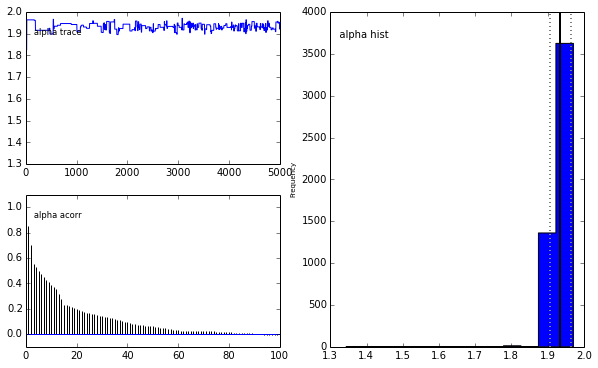Second, using continuous data with $\alpha=3$.

In :
test = powerlaw.Power_Law(xmin = 1., parameters = [3.], discrete=False)
simulated = test.generate_random(5000)
analyze(simulated, discrete=False)

 [-----------------100%-----------------] 5000 of 5000 complete in 1.4 sec
2.93062043077
Plotting alpha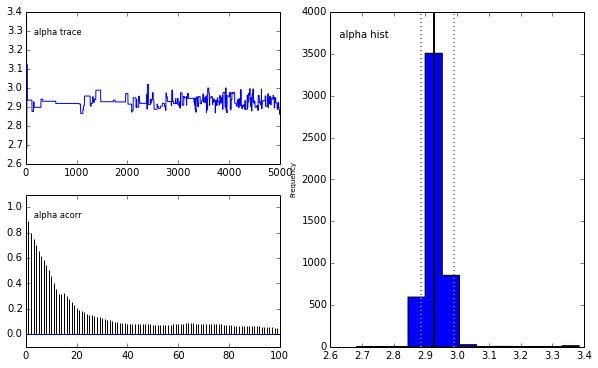In both examples, we can clearly see that the posterior distributions of the $\alpha$ values are very certain in regard to the value of the parameter which is very similar to the initital parameter set when generating the random power law data.

Next, we will evaluate our approach by also applying it to some examples used in Aaron Clauset's famous power law paper (http://arxiv.org/abs/0706.1062). The data can be found at http://tuvalu.santafe.edu/~aaronc/powerlaws/data.htm.

We begin by using the classic Moby Dick word frequency data.

In :
import urllib

data = list()
for line in urllib.urlopen("http://tuvalu.santafe.edu/~aaronc/powerlaws/data/words.txt"):
data.append(line.strip())
analyze(data)

 [-----------------100%-----------------] 5000 of 5000 complete in 3.8 sec
1.77287396672
Plotting alpha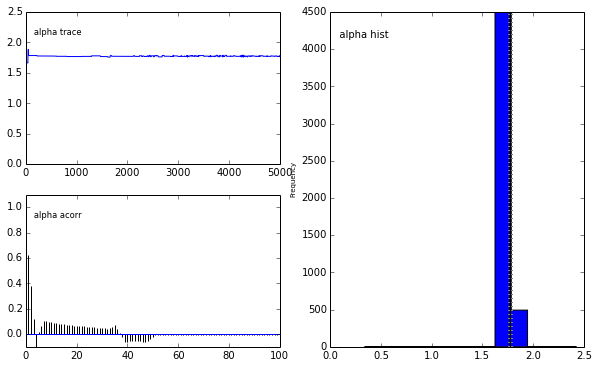In Clauset et al. the authors find a value for $x_{min} = 7$ and $\alpha = 1.95$. As we currently do not have a working function to also determining the best $x_{min}$ value, we will set the same $x_{min}$ value and find the appropriate $\alpha$ value. We end up with close to the exact same $\alpha$ of around $1.95$.

In :
analyze(data,xmin=7)

 [-----------------100%-----------------] 5000 of 5000 complete in 1.6 sec
1.9600505014
Plotting alpha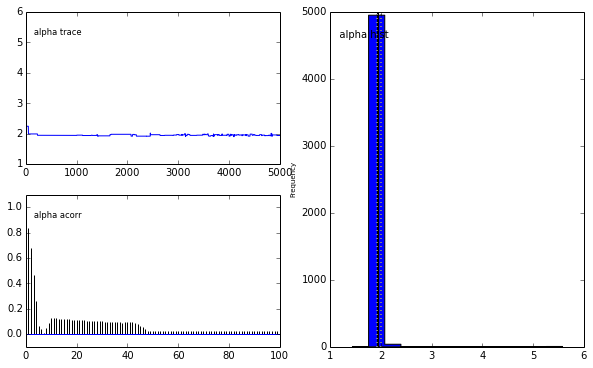Finally, we use a dataset capturing the size of acres of wildfire (this time continuous) occurring in the US between 1986 and 1996.

In :
data = list()
for line in urllib.urlopen("http://tuvalu.santafe.edu/~aaronc/powerlaws/data/fires.txt"):
data.append(line.strip())
analyze(data, discrete=False)

 [-----------------100%-----------------] 5000 of 5000 complete in 12.9 sec
1.48610494777
Plotting alpha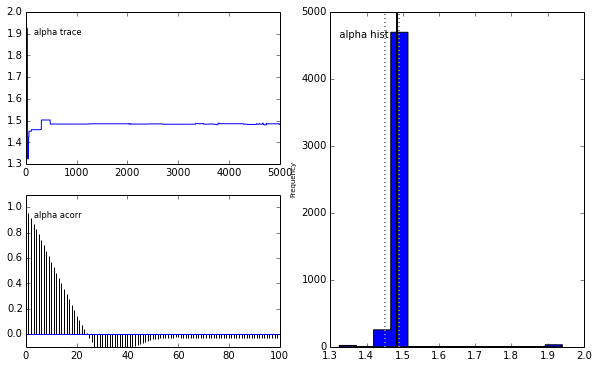Again, Clauset et al. find a much higher $x_{min}$ value of $6324$. Hence, we set $x_{min}$ to this value and again apply the MCMC process. The posterior distribution of $\alpha$ again resembles the value found by Clauset et al. -- $\alpha=2.2$.

In :
analyze(data, discrete=False, xmin=6324.)

 [-----------------100%-----------------] 5000 of 5000 complete in 4.0 sec
2.16554447932
Plotting alpha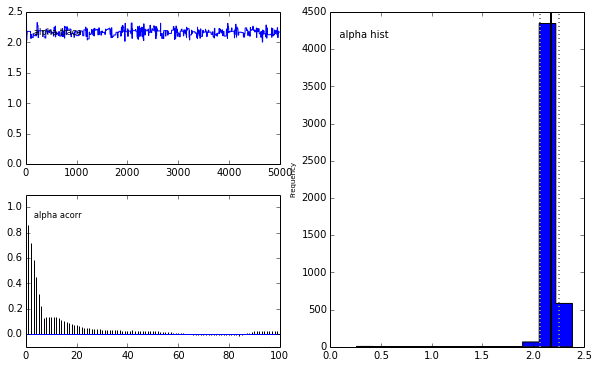### Whats next?

First of all, I want to incorporate the process of also determining the appropriate $x_{min}$ value. If anyone has a hint or idea of how to solve this, please let me know. Furthermore, I want to analyze the goodness-of-fit and also compare the power law distribution to other candidate distributions like the Weibull distribution. This is a crucial step needed for gaining insights into the underlying processes of the data. I previously blogged about this issue at http://www.philippsinger.info/?p=247.